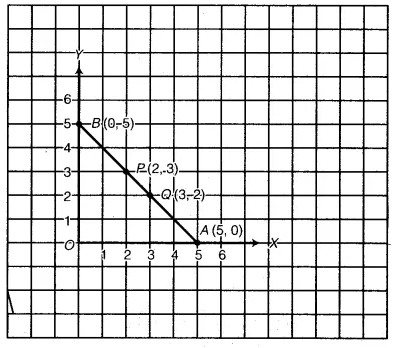# Draw the line passing through (2, 3) and (3, 2). Find the coordinates of the points

Draw the line passing through (2, 3) and (3, 2). Find the coordinates of the points at which this line meets the x-axis and y-axis.

Draw the x-axis and y-axis. Plot the points P(2, 3) and Q(3, 2). Join PQ and produce PQ on both sides to meet the x-axis at A and y-axis atB. Clearly, the coordinates of A and B are (5, 0) and (0, 5) respectively.